# Ravi

•### Interactive Parabola - Vertex Form

Activity

Ravi

•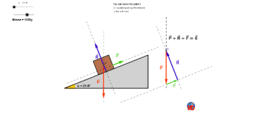### Copy of Inclined plane

Activity

Ravi

•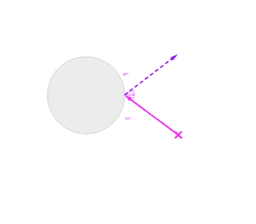### Reflection off Round Surface - Example 2

Activity

Ravi

•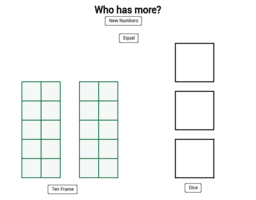### VG Number and Representation Game

Activity

Ravi

•### CT Find the Point

Activity

Ravi

•### TG Solution of a pair of linear equations

Activity

Ravi

•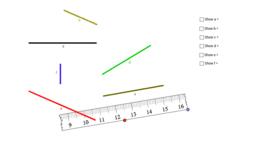### Copy of Broken Centimeter Ruler

Activity

Ravi

•### TG Consistency of linear equations Coincident lines

Activity

Ravi

•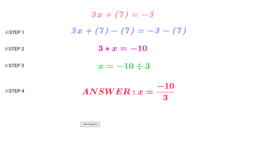### TG Linear Equations of the form ax+b=c. Level 1.

Activity

Ravi

•### TG Visual soln of a sys of two lin eqns

Activity

Ravi

•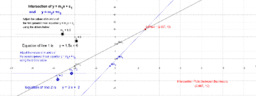### TG Intersection between 2 straight lines

Activity

Ravi

•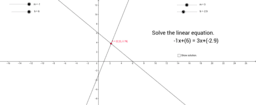### TG Solving linear equations #2

Activity

Ravi

•### TG Visualizing a System of Linear Equations

Activity

Ravi

•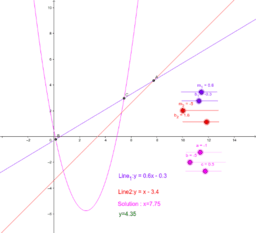### TG Visualizing a System of Linear Equations

Activity

Ravi

•### TG Visualizing a System of Linear Equations

Activity

Ravi

•### TG system of 2 lin eqns

Activity

Ravi

•### TG Workbench for Pair of Lin Eqns in Two Var

Activity

Ravi

•### TG rat and irrat

Activity

Ravi

•### Copy of Prime Factorization

Activity

Ravi

•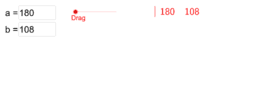### TG HCF & LCM of Two Numbers by Short Division

Activity

Ravi

•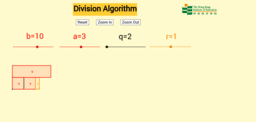### TG Division Algorithm

Activity

Ravi

•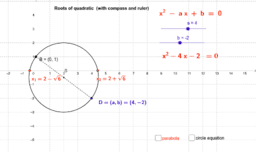### TG Roots of quadratic (with compass and ruler)

Activity

Ravi

•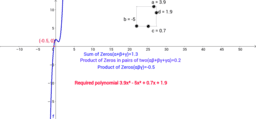### TG Cubic Polynomial

Activity

Ravi

•Activity

Ravi